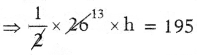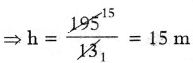# AP Board 7th Class Maths Solutions Chapter 11 Area of Plane Figures Ex 11.1

SCERT AP 7th Class Maths Solutions Pdf Chapter 11 Area of Plane Figures Ex 11.1 Textbook Exercise Questions and Answers.

## AP State Syllabus 7th Class Maths Solutions 11th Lesson Area of Plane Figures Ex 11.1

Question 1.
Calculate the area of the following triangles given below:
(a)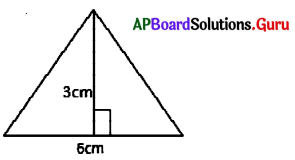From the figure, base = 6 cm
Height = 3 cm
Area of the triangle = $$\frac{1}{2}$$ ∙ b ∙ h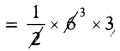= 9 sq.cm(b)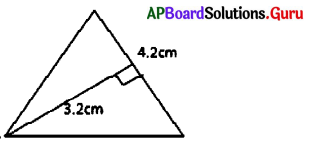From the figure, base = 4.2 cm
Height = 3.2 cm
Area of the triangle = $$\frac{1}{2}$$ ∙ b ∙ h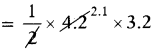= 6.72 sq.cm

(a)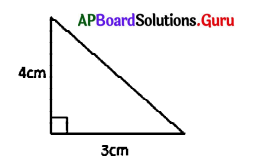From the figure, base = 3 cm
Height = 4 cm
Area of the triangle = $$\frac{1}{2}$$ ∙ b ∙ h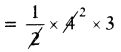= 6 sq.cm

(a)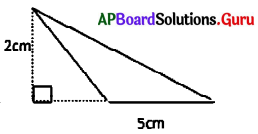From the figure, base = 5 cm
Height = 2 cm
Area of the triangle = $$\frac{1}{2}$$ ∙ b ∙ h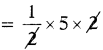= 5 sq.cmQuestion 2.
Find the area of triangle with base 3.8 cm and height 4.6 cm.
Given base of the triangle b = 3.8 cm
Height of the triangle h = 4.6 cm
Area of the triangle = $$\frac{1}{2}$$ ∙ b ∙ h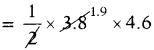∴ Area of the triangle = 8.74 sq.cm.

Question 3.
The surface area of a triangular shape window is 24 sq.m, and height is 6m., then find the base of the triangle, if the cost of glass fitting per sqm is ₹ 250, then find total cost of glass fitting for window.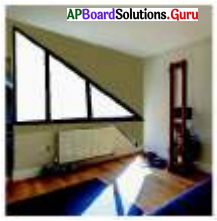Let,
base of the triangular window = b m
Height of the triangular window h= 6m
Area of triangular window = $$\frac{1}{2}$$ ∙ b ∙ h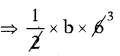= 24 sq.cm
⇒ 3b = 24
⇒ b = $$\frac{24}{3}$$
= 8
∴ Base of the triangular window = 8m
Cost of glass fitting per sq.m = ₹ 250
Cost of glass fitting per 24 sq.m = 250 × 24
∴ Total cost of glass fitting for window = ₹ 6000/-Question 4.
A traffic signal plate in the shape of triangle is having base 20 cm, height 15 cm. Find the area of the triangle. If the cost of painting is ₹ 2 per Sq.cm, then find the total cost for painting the signal board on one side.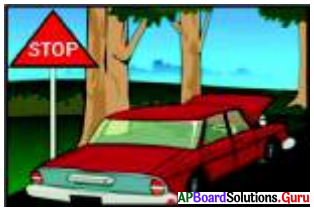Given the base of the signal plate b = 20 cm
Height of the signal plate h = 15 cm
Area of the signal plate = $$\frac{1}{2}$$ ∙ b ∙ h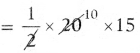= 150 sq.cm
∴ Area of the signal plate = 150 sq.cm
Cost of painting per sq.cm = ₹ 2
Cost of painting for 150 sq.cm = 150 × 2
∴ Total cost for painting the signal board = ₹ 300

Question 5.
find the area of triangular shaped wall painting whose base is 24m, height is 38m. Find the area of the triangle, if the cost of painting is ₹ 50 per Sq. m. and also find the total cost of painting.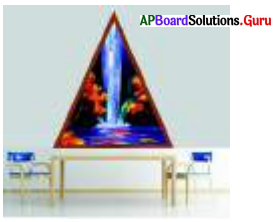Given base of the triangular wall painting = 24 m Height = 38 m
Area of wall painting = $$\frac{1}{2}$$ ∙ b ∙ h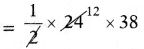= 456 sq.m.
Cost of painting per 1 sq.m = ₹ 50
Cost of painting for 456 sq.m = 456 × 50
∴ Total cost for painting per 456 sq.m = ₹ 22,800Question 6.
The area of triangle-shaped elevation of a house is 195 Sq.m. Its base is 26m. Find the height of elevation. Find the total cost of cementing, if the cost of cementing per Sq.m, is ₹ 250.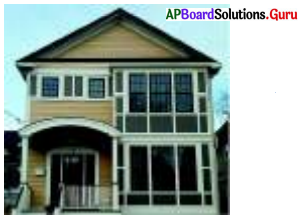= $$\frac{1}{2}$$ ∙ b ∙ h